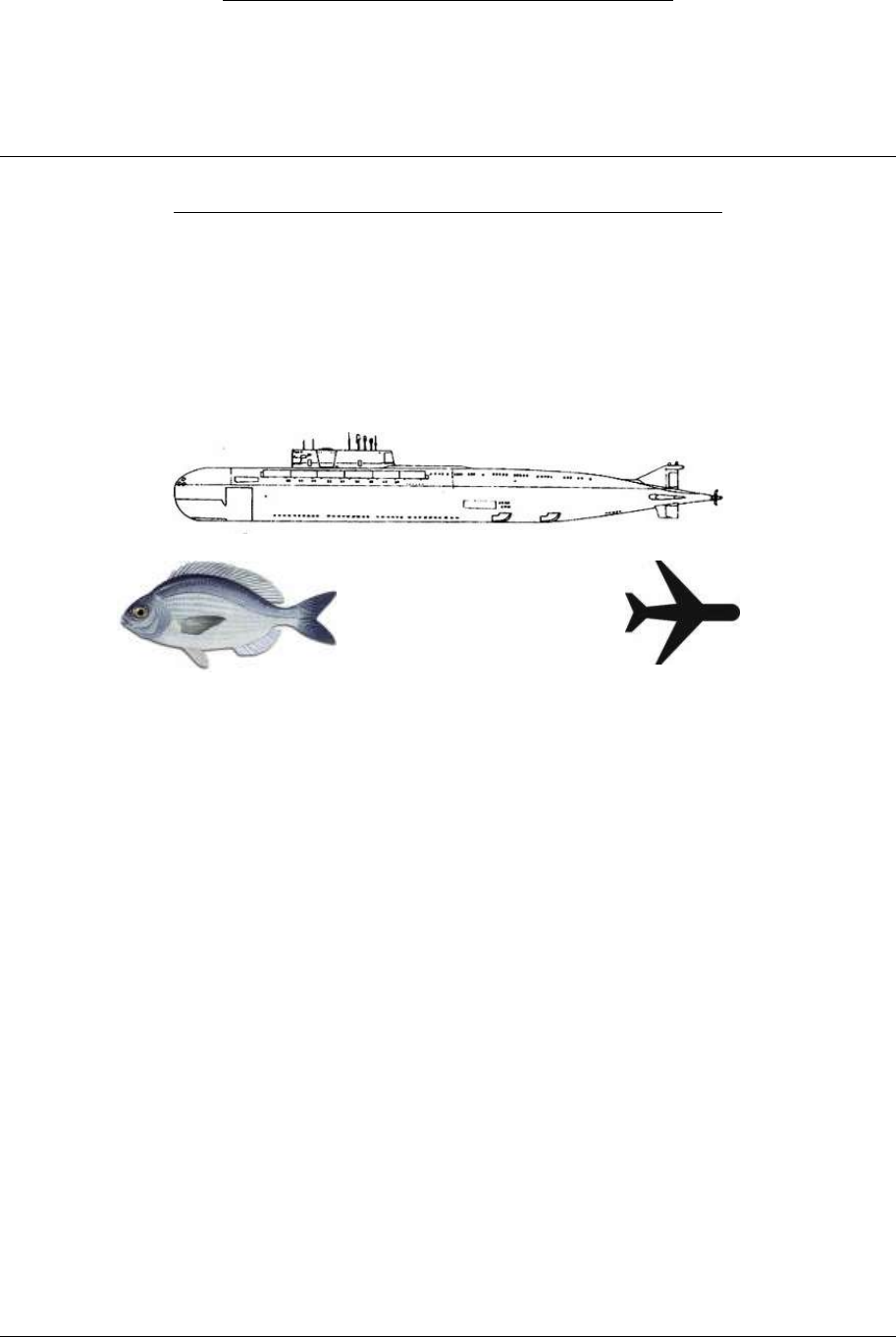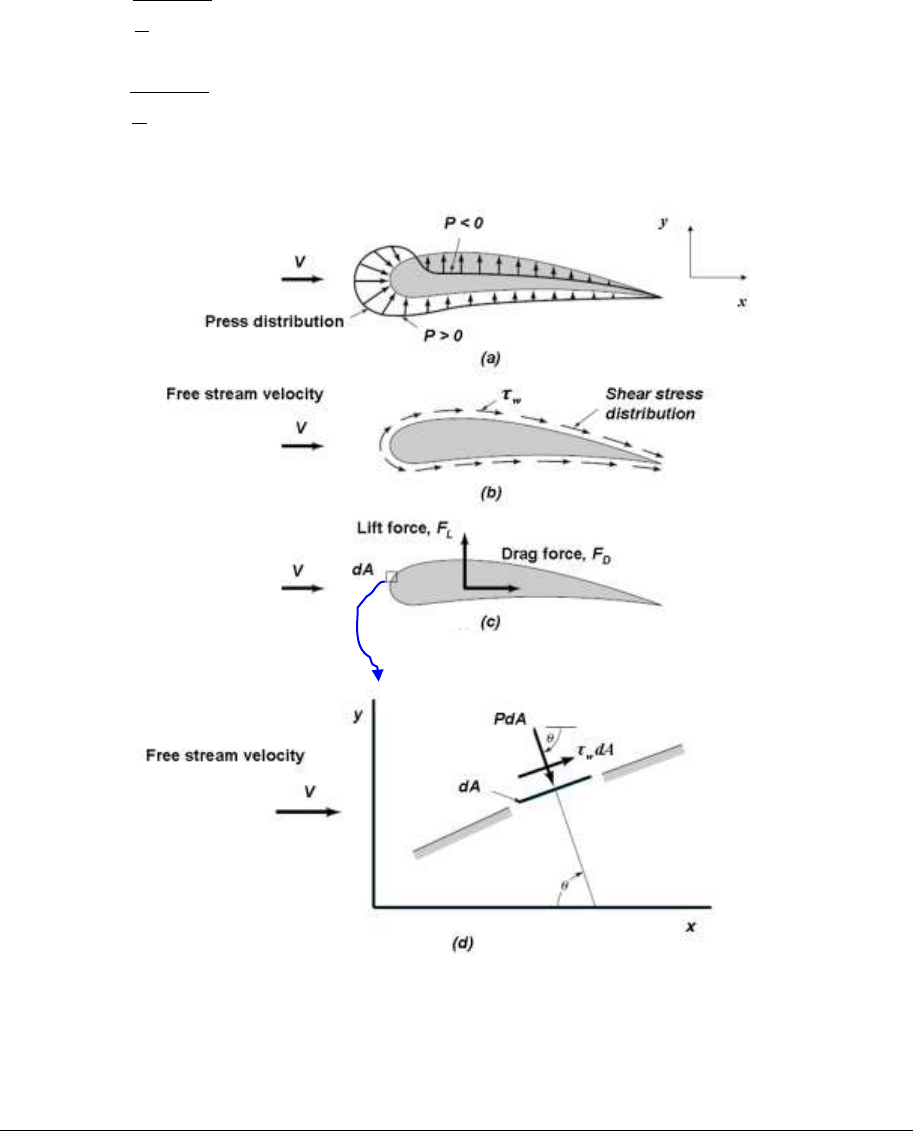# ENGR2000 Lecture Notes - Lecture 8: John Wiley & Sons, Terminal Velocity, Stagnation Point

28 views17 pages
School
Department
Course
ProfessorPrepared by Dr Hongwei Wu Chapter 8 Page 1 of 17
2nd Year Fluid Mechanics, Faculty of Engineering and Computing, Curtin University
ENGR2000: FLUID MECHANICS
For Second-Year Chemical, Civil and Mechanical Engineering
FLUID MECHANICS LECTURE NOTES
CHAPTER 8 FLOW OVER IMMERSED BODY
8.1 Introduction
We have discussed pipe flow in Chapter 6, which is internal flow because the fluid is
confined within well-defined boundaries. This chapter deals with external flows, i.e. flows
over bodies immersed in the fluid. Typical examples of external flows include the flow of
water over a submarine (Figure 8-1a) or a fish (Figure 8-1b), the flow of air over an aircraft
(Figure 8-1c).
(a)
(b)
(c)
Figure 8-1 Examples; a) submarine; b) fish; c) aircraft;
The fluid forces (drag and lift) on the immersed bodies are of important considerations in
practice. In this chapter, we will learn the fundamentals of drag and lift, as well as the
methods for determining and optimising these forces in engineering applications.
8.2 Drag and lift
As shown in Figure 8-2, the forces exerted on the surface of an aerofoil by the fluid include
the pressure force (Figure 8-2a) and the viscous force (Figure 8-2b). The results of these
forces are the net drag force FD and lift force FL (Figure 8-2c). Taking a small element dA on
the aerofoil surface in Figure 8-2c, we can decompose the pressure force (PdA) and viscous
force (
dA
w
) into x-direction force dFx and y-direction force dFy, as shown in Figure 8-2d,
.
Integrating dFx and dFy along the surface, we can calculate the drag and lift forces as,
sincos
(E8-1)
cossin
. (E8-2)
find more resources at oneclass.com
find more resources at oneclass.com
Unlock document

This preview shows pages 1-3 of the document.
Unlock all 17 pages and 3 million more documents.Prepared by Dr Hongwei Wu Chapter 8 Page 2 of 17
Equations E8-1 and E8-2 reveals that 1) both shear stress and pressure contribute to the drag
and lift. In the case of drag, the former is called friction drag; the later is called pressure drag;
2) to calculate drag and lift, sufficient knowledge are required on three aspects: the body
shape as it determines the distribution of
; the distribution of pressure P and the distribution
of shear stress
w
along the surface.
Practically, it is very difficult to obtain the distribution of pressure and shear stress. Therefore,
E8-1 and E8-2 are generally not very useful. To make it easy for engineers, dimensionless
coefficients are often used instead. These dimensionless numbers are called drag coefficient
CD and lift coefficient CL, defined as
AV
F
CD
D
2
2
1
(E8-3)
AV
F
CL
L
2
2
1
(E8-4)
where A is projected (or frontal) area, i.e. the project area of the body in the flow direction.
Figure 8-2 Forces on an aerofoil : a) pressure force; b) viscous force; c). resultant drag and
lift; d) pressure and viscous forces on a small surface area dA in (c), plotted in an x-y
coordination system.
find more resources at oneclass.com
find more resources at oneclass.com
Unlock document

This preview shows pages 1-3 of the document.
Unlock all 17 pages and 3 million more documents.Prepared by Dr Hongwei Wu Chapter 8 Page 3 of 17
8.3 The boundary layer
8.3.1 Hydraulician and hydrodynamicist
For a fundamental understanding on the cause of drag and lift, we must have a good
understanding on the boundary layer concept. Let’s start with some historical background on
two groups of fluid mechanicians who developed two different approaches in dealing with
problems of fluid mechanics by late 19th century.
One is the group of hydraulicians, focused on experiments and attempted to generalise useful
design equations from experimental data. This group developed the filed of experimental
hydraulics, delivering empirical solutions with little theoretical content. The other is the group
of hydrodynamicists, who focused on differential equations describing flows and tried to
apply them to practical problems. In order to solve these differential equations, the fluid was
assumed to have zero viscosity and constant density. This group developed the filed of
theoretical hydrodynamics, seeking pure theoretical solutions based on ideal-fluid flows.
The ideal-fluid solutions of hydrodynamicists agreed well with the observations of flows did
not involve solid surface, e.g. tides, however did not agree with observed behaviours in the
problems that concerned the hydraulicians, e.g, flow over immersed bodies. The following are
two typical examples.
(1) Flow over a thin plate
Figure 8-3 illustrates the differences between the ideal solutions by the hydrodynamicists and
the experimental observations by the hydraulicians for flow over a thin plate. For an ideal-
fluid flow (Figure 8-3a), the fluid is inviscid, there is no friction between the fluid and the
surface of the thin plate. Therefore, the fluid will maintain its free stream velocity when it
flows over the thin plate. However, for a real-fluid flow, the interaction between the viscous
fluid with the surface leads to a velocity gradient near the surface of the plate although the
fluid far away from the plate still maintains its free stream velocity.
(2) Flow over a circular cylinder
Figure 8-5 shows the patterns of ideal and real flow over a circular cylinder. In absence of
viscous effects, the wall shear stress is zero therefore the streamlines are symmetrical (see
Figure 8-6a). The fluid coasts from the front (point A), pasts the top (point C) and then
reaches to the rear (point F) of the cylinder.
However, the experimental observations by the hydraulicians are very different from the
above analysis by the hydrodynamicists, as shown in Figure 8-4b. A real-fluid flow cannot
coast along the cylinder surface down to point F. Instead, flow separation occurs from the
surface at a point between point C and F, leading to the formation of turbulent wake in the
downstream (see Figure 8-4b). The friction between the viscous fluid and the cylinder surface
leads to inevitable energy loss so that the flow does not have sufficient kinetic energy to travel
along the surface down to the rear of the cylinder.
From the above examples, it seems that the hydrodynamicists calculated what can NOT be
observed while the hydraulicians observed what can NOT be calculated . By early 20th
century, the hydraulicians and hydrodynamicists had completely gone to live in their own
worlds. The hydraulicians continued to solve their problems by trial and error based on
experiments while the hydrodynamicists kept publishing academic papers based on
mathematics with little bearing on engineering problems.
find more resources at oneclass.com
find more resources at oneclass.com
Unlock document

This preview shows pages 1-3 of the document.
Unlock all 17 pages and 3 million more documents.